Circles
Area of 2D shapes
Composite Figures
Volume
Surface Area
100

Area? (π = 3.14)153.86 in2

100

What is the area of a triangle with a base of 20 cm and a height of 6cm?

60 cm2

100

Area of the shaded region?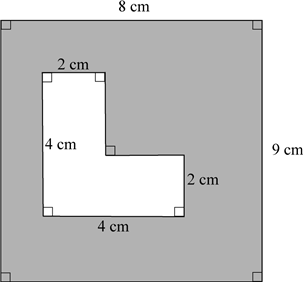60 cm2

100343 in3

100

What is the surface area (all dimensions in inches)?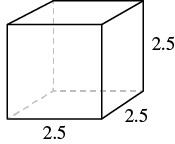37.5 in2

200

Circumference? (π = 3.14)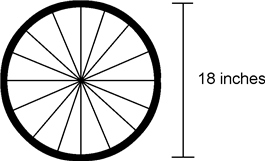56.52 in

200

Find the area: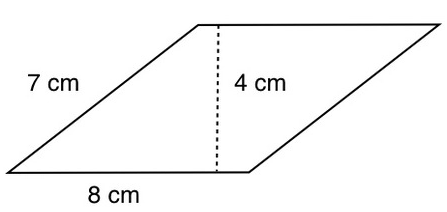32 cm2

200

Find the area of the shaded portion.  Use π = 3.1450.24 cm2

200

What is the Volume?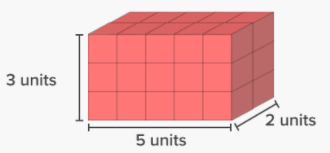30 units3

200

What is the surface area?684 inches2

300

What is the diameter of a circle that has an area of 78.5 meters2

10 m

300

Area of a trapezoid with b1 = 15 inches, b2 = 7 inches, and the height is 16 inches?

176 in2

300

Find the area.  π = 3.14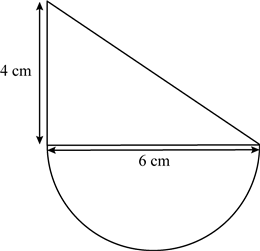26.13 cm2

300

Find the volume1848 m3

300

What is the surface area?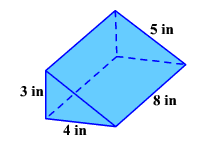108 in2

400

What is the radius of a circle that has a circumference of 81.64 inches?

13 inches

400

Area of this trapezoid (all units are cm)?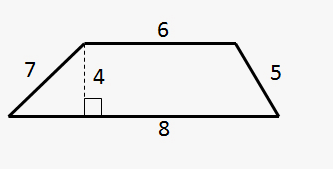28 cm2

400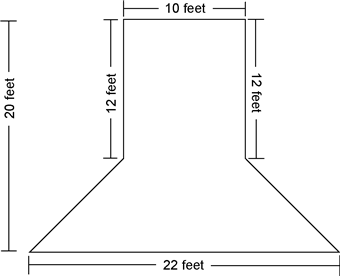248 ft2

400

Find the volume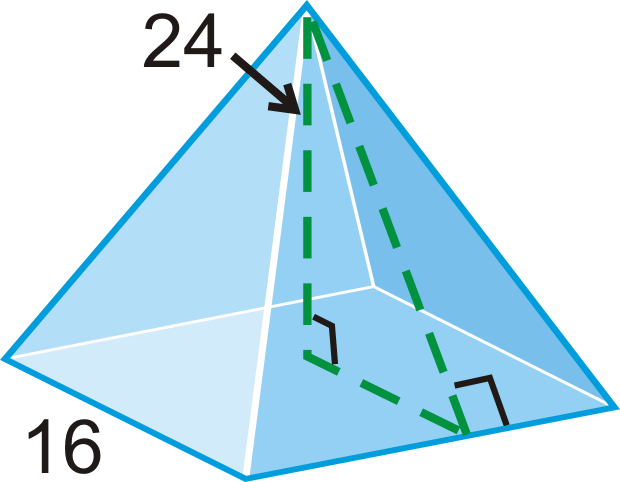2048 units3

400

What is the surface area?16 ft2

500

Area? (π = 3.14)628 ft2

500

What is the area of the shaded triangle?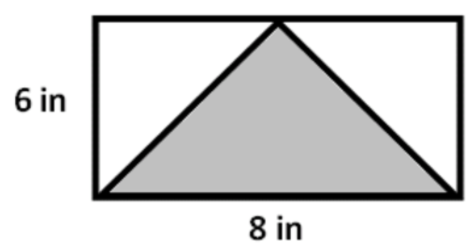24 in2

500

If the radius of the pond is 5 feet, how much area is the garden? (π = 3.14)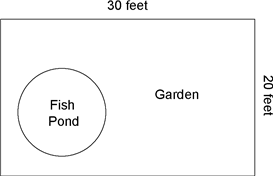521.5 feet2

500

Find the volume:471 in3

500

Eduardo is building a mailbox. The mailbox will be 10 inches wide, 20 inches deep, and 12 inches tall.

What is the surface area of the mailbox?

1120 in2

Click to zoom